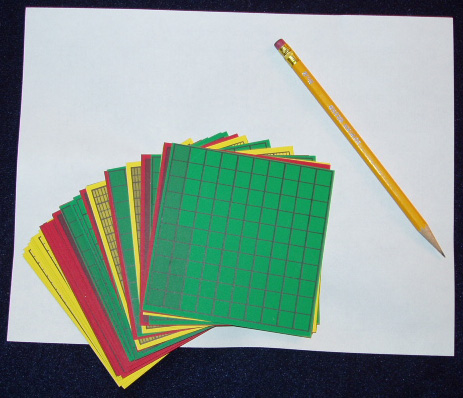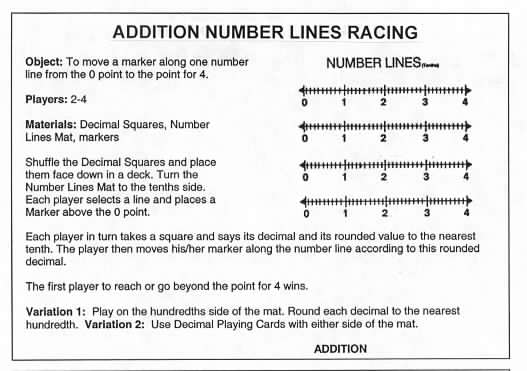Home
 Creating Number Lines . Materials: A deck of Decimal Squares for each group and a pencil and sheet of paper for each person.. The number line is an important concept in mathematics and is frequently used to teach and illustrate decimals. However the number line model is more abstract than models such as Base Ten Pieces and Decimal Squares which can be picked up and manipulated. The following sequence of activities connects the Decimal Squares model to creating a number line and linear measure. Ask each person to select a red square and carry out the following steps (see illustration below): 1. Turn a standard sheet of paper lengthwise and place the square near the left edge as shown below. 2. Use the bottom edge of the square to draw a line and place a mark on the line for each of the 11 vertical lines of the square. 3. Label the left end point 0 the right end point 1 and write the decimal for the square under the mark which is at the end of the shaded part of the square. For example this is .3 for the square below. Place other decimal squares above the line and write the decimal for their shaded amounts. 4. When the unit length from 0 to 1 has been labeled with decimals for tenths measure the length of some small objects such as an eraser Decimal Playing Card etc. Discuss the use of decimals to tell what part of a region is shaded (area) or to tell the distance (length) a point is from the zero point.This activity can be continued for measuring larger objects by using red squares to draw lines of length 2 units units etc. (see below) and labeling the mixed decimals for the tenths. This activity provides for a gradual transition to the use of decimals for measuring length. Using the Decimal Squares model to create a number line from 0 to 2 for measuring in tenths.NUMBER LINES RACING There are two versions of this game one for addition (see rules below) and one for subtraction. These games are on pages 83 and 87 of the Decimal Squares Teacher’s Guide. Show a transparency of the Number Lines from the end pages of the Decimal Squares Teacher’s Guide and briefly illustrate one or two moves in the game.